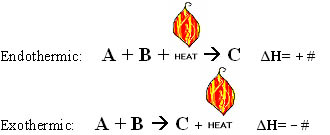## Thermochemical Equations

#### Learning Objective

• Give examples of thermochemical equations

#### Key Points

• If $\Delta H$ is negative, the reaction is exothermic; if $\Delta H$ is positive, the reaction is endothermic.
• Thermochemical equations have the general form: $A+B\rightarrow C, \Delta H=(\pm n)$ .
• The value of enthalpy is dependent on the reaction conditions, as well as the concentration of reactants and products.

#### Terms

• thermochemical equationA special equation type, denoting the overall change in energy.
• enthalpyIn thermodynamics, a measure of the heat content of a chemical or physical system.
• Hess’s lawStates that the enthalpy change for a reaction is the same whether it occurs in one step or in a series of steps.

Enthalpy (H) is a measure of the energy in a system, and the change in enthalpy is denoted by $\Delta H$. Since enthalpy is a state function, the value of $\Delta H$ is independent of the path taken by the reactions to reach the products. Values of $\Delta H$ can be determined experimentally under standard conditions.

A thermochemical equation is a balanced stoichiometric chemical equation which includes the enthalpy change. The equations take the form: $A+B\rightarrow C,\: \Delta H =(\pm n)$

## Thermochemical Equations for Endothermic Reactions

The sign of the $\Delta H$ value indicates whether or not the system is endothermic or exothermic. In an endothermic system, the $\Delta H$ value is positive, so the reaction absorbs heat into the system. The equation takes the form:

$heat+A+B\rightarrow C,\;\Delta H=+$

Notice that in an endothermic reaction like the one depicted above, we can think of heat as being a reactant, just like A and B.

## Thermochemical Equations for Exothermic Reactions

In an exothermic system, the $\Delta H$ value is negative, so heat is given off by the reaction. The equation takes the form:

$A + B \rightarrow C + heat,\: \Delta H = -$

Notice that here, we can think of heat as being a product in the reaction.

$\Delta H$ is dependent on both the phase (solid, liquid, or gas) as well as the molar ratios of the reactants and products. Therefore, all thermochemical equations must be stoichiometrically balanced. This becomes important once we begin working with Hess’s law.Thermochemical equationsThermochemical equations can describe endothermic or exothermic reactions.